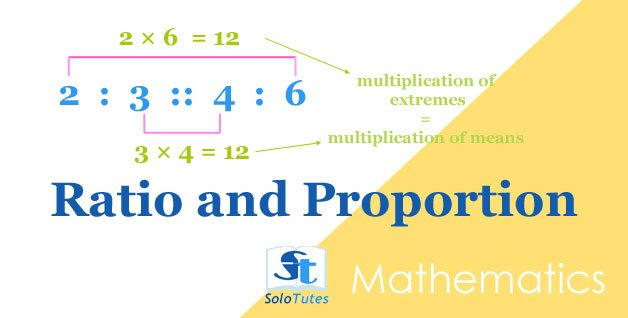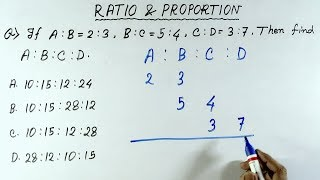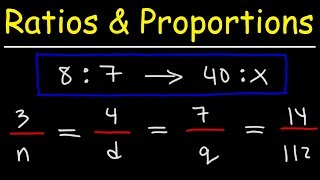SoloTutes

Search By Topics

Followers ×
##### ratio and proportion
Lessonsolotutes •
7 Apr 2021## Ratio and Proportion, Basic Tricks and Practice Problems

ratio  can be written in the form of a :b ( where a and b are integers) is the simplified fractional relation ( a/b)  between two quan

Quizsolotutes •
7 Apr 2021

## Ratio

Solve these practice problems on ratio and proportion.

1 of 3 〉 What number has 5 to 1 ratio to the number 10?
• 42
• 55
• 50
• 60
2 of 3 〉 There are 120 students in a picnic tour, if ratio of girls and boys is 5 : 7 then how many boys are in this tour?
• 50
• 70
• 55
• 120

1 more 👇

### Videos ❯

🗘
Share on
##### Ratio and Proportion Word Problems - Math

This math video tutorial provides a basic introduction into ratio and proportion word problems. Here is a list of examples and practice problems: My Website: ...
Reference ❯ Ratio and Proportion, Basic Tricks and Practice Problems

### Similar Videos ❯##### Aptitude Made Easy - Ratio

Get the latest interview tips,Job notifications,top MNC openings,placement papers and many more only##### Ratios and Proportions With Word Problems!

This math video review tutorial explains how to use ratios and proportions to solve word problems. Y##### Ratio and Proportion Questions with Tricks | Maths | SYNDICATE, IBPS CLERK MAINS, SSC CHSL, SSC CGL

In this video we shall discuss Ratio and Proportion Questions with Tricks For SYNDICATE, IBPS CLERK##### Ratio and Proportion shortcut tricks in hindi | Ratio and proportion tricks | ratio and proportion

Ratio##### Ratio and Proportion Tricks | Ratio and proportion Concept/Trick/Method in Hindi | CAT, UPSC, CTET

In this video we will learn how to solve Ratio and proportion question in a very quick way so that y

### More Videos ❯##### Ratio and Proportion Word Problems - Math

This math video tutorial provides a basic introduction into ratio and proportion word problems. Here##### Ratio and Proportion shortcut tricks in hindi | Ratio and proportion tricks | ratio and proportion

Ratio##### Ratio and Proportion Tricks | Ratio and proportion Concept/Trick/Method in Hindi | CAT, UPSC, CTET

In this video we will learn how to solve Ratio and proportion question in a very quick way so that y##### Ratio and Proportion Word Problems - Math

This math video tutorial provides a basic introduction into ratio and proportion word problems. Here

!Disclaimer:The videos displayed above are dynamically synced using youtube search api as per the content of this page and are for educational purposes only. We are not the creater of any videos displaying here. The credits and rights goes to the respective creaters/channel-owners on Youtube. .

### Do you Want to Write Academic Notes Here?

Join SoloTutes.com Telegram Channels@pharmacy_exams@nurseexams@solotutes
##### Visual Stories